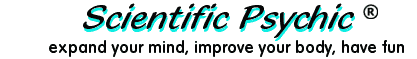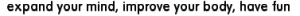Index# The Chicken and Leg Problem

A chicken farmer also has some cows for a total of 30 animals, and the animals have 74 legs in all. How many chickens does the farmer have?

This is a typical algebra problem with two unknowns, and we need to have two equations to solve the problem. Let X be the number of cows, and Y the number of chickens.

The number of cows plus the number of chickens equals 30:
X + Y = 30
Since cows have four legs and chickens have two legs, we can also have an equation for the number of legs.
4 X + 2 Y = 74
Rearranging the first equation we get
X = 30 - Y
Substituting X in the second equation gives us
4 × (30 - Y) + 2 Y = 74

120 - 4 Y + 2 Y = 74

-2 Y = 74 - 120 = -46

Y = 23
The farmer has 23 chickens. (and 7 cows).

Tim, a good-humored reader who probably got the wrong answer, sent the following comment:

I believe you haven't looked at the question as closely as you thought. Your answer is forgetting about the farmer's legs. So your total would be 76 total legs, the correct answer I believe is 24 chickens, which in the end put you to 72 total legs between the animals and plus the 2 farmer's legs get you to 74.

What Tim does not know is that this farmer has a wife, and a centipede lives in the basement. So Tim's answer is off by at least 100.